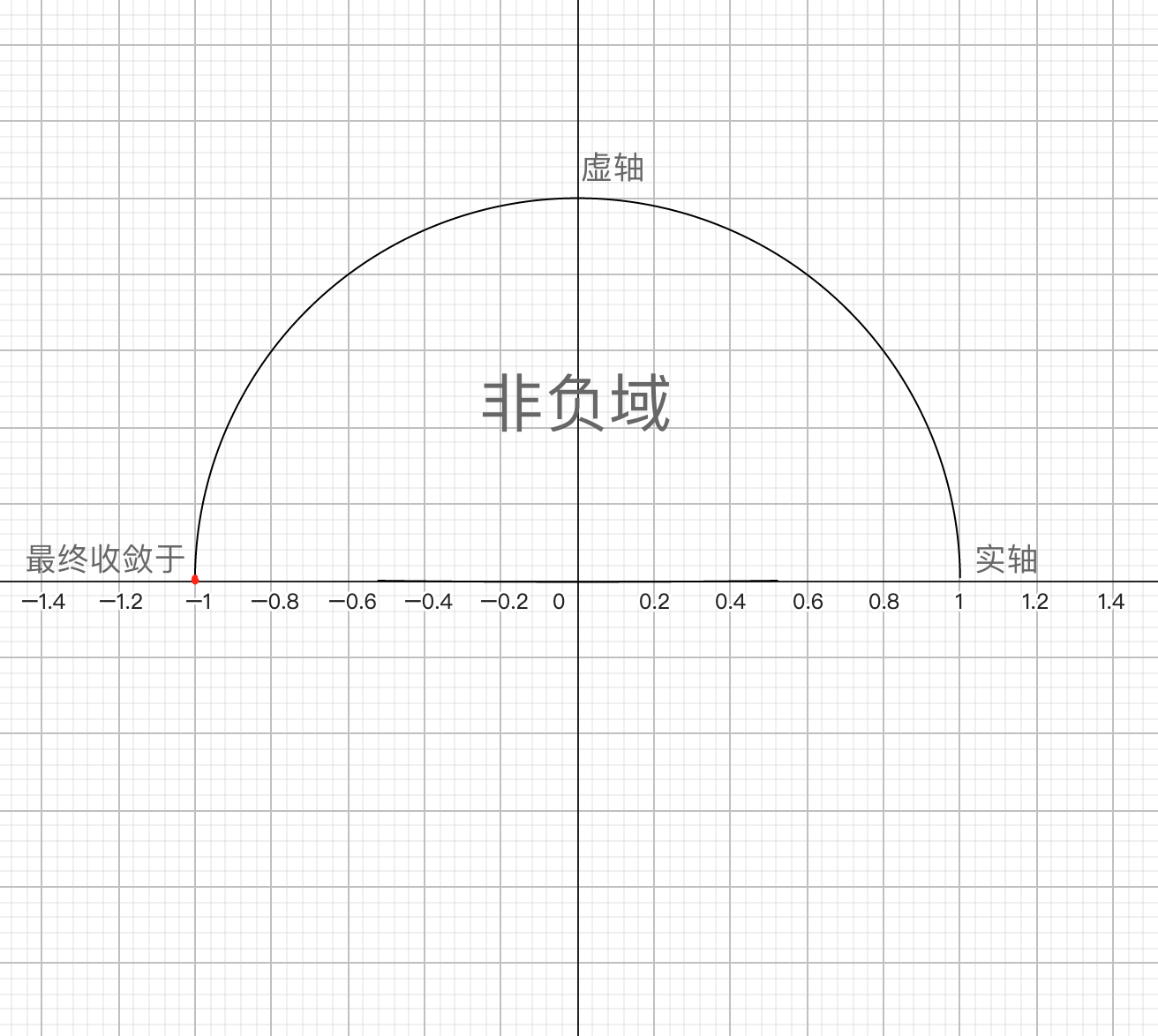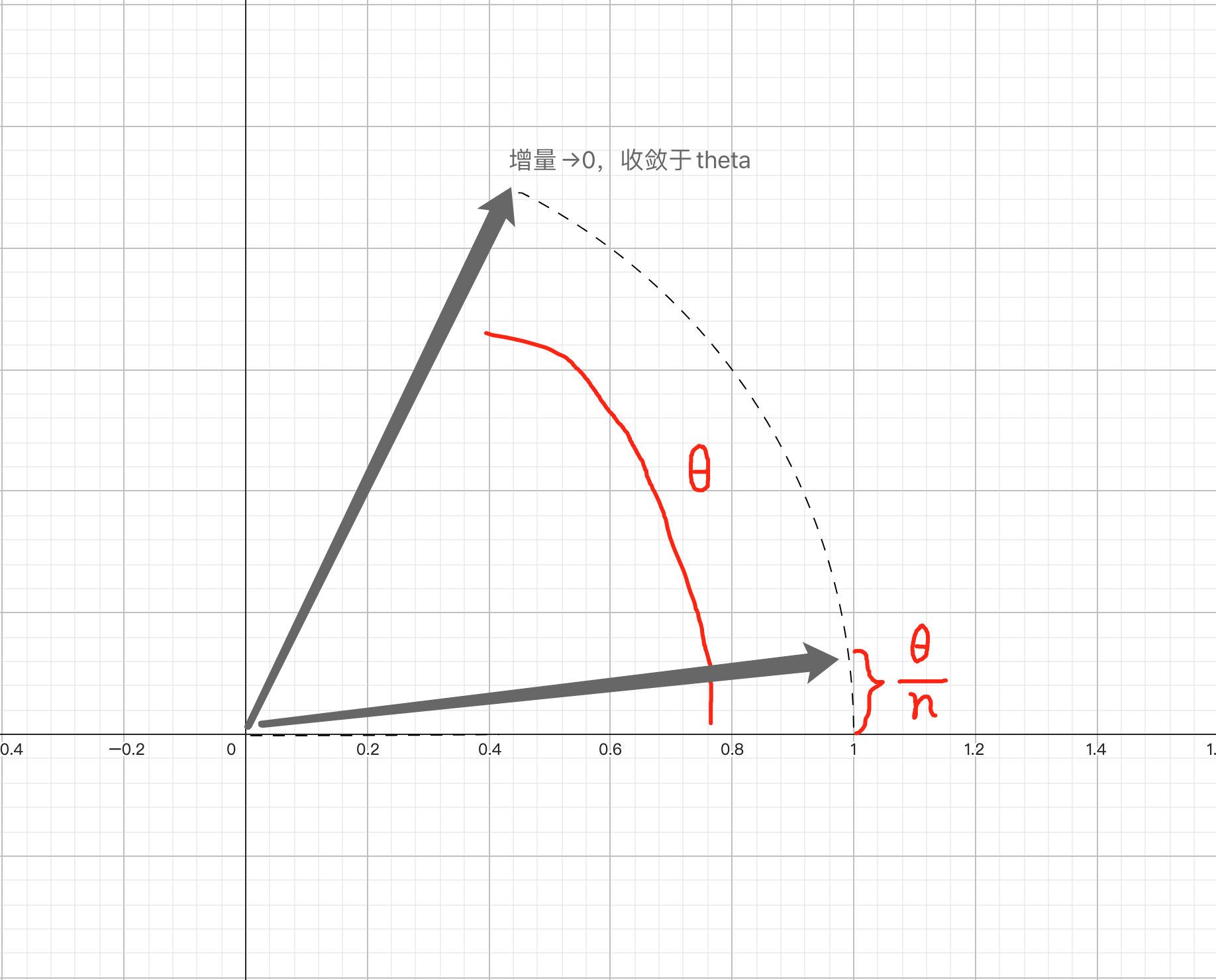# 欧拉公式的一般式推广：

Author：张一极

date：2022年11月13日08:41:53

a.先验知识：定义$e=\underset{n\to \mathrm{\infty }}{lim}{\left(1+\frac{1}{n}\right)}^{n}$$e=\lim _{n \rightarrow \infty}\left(1+\frac{1}{n}\right)^n$ from 维基百科

$\begin{array}{}\text{(1)}& \begin{array}{c}\because {e}^{\mathrm{ln}{\left(1+\frac{x}{n}\right)}^{n}}={e}^{n\mathrm{ln}\left(1+\frac{x}{n}\right)}\end{array}\end{array}$

$\begin{array}{}\text{(2)}& \therefore \underset{n\to +\mathrm{\infty }}{lim}{\left(1+\frac{x}{n}\right)}^{n}=\underset{n\to +\mathrm{\infty }}{lim}{e}^{n\mathrm{ln}\left(1+\frac{x}{n}\right)}\end{array}$

$\begin{array}{}\text{(3)}& \begin{array}{c}\\ \therefore {e}^{\underset{n\to +\mathrm{\infty }}{lim}\mathrm{ln}\left(1+\frac{x}{n}\right)}={e}^{\underset{n\to +\mathrm{\infty }}{lim}\mathrm{ln}\left(1+\frac{x}{n}\right)\frac{1}{n}}\end{array}\end{array}$

$\begin{array}{}\text{(4)}& \text{原}\text{式}=\underset{n\to +\mathrm{\infty }}{lim}\frac{\left(\frac{-x}{{n}^{2}}\right)\frac{1}{1+\frac{x}{n}}}{-\frac{1}{{n}^{2}}}\end{array}$

TO:↓

$\begin{array}{}\text{(5)}& \therefore \text{原}\text{式}\text{化}\text{简}\text{后}={e}^{\underset{n\to +\mathrm{\infty }}{lim}\frac{x}{1+\frac{x}{n}}}={e}^{x}\end{array}$

$\begin{array}{}\text{(6)}& \underset{n\to \mathrm{\infty }}{lim}{\left(1+\frac{x}{n}\right)}^{n}={e}^{x}\end{array}$

$\begin{array}{}\text{(7)}& {e}^{i\pi }=\underset{n\to \mathrm{\infty }}{lim}{\left(1+\frac{i\pi }{n}\right)}^{n}\end{array}$

$n\to \mathrm{\infty }$$n \rightarrow \infty$时，幅角增量为0， 由于${\left(1+\frac{i\pi }{n}\right)}^{n}$$\left(1+\frac{i \pi}{n}\right)^n$为非负数，故其将收敛在到达非负域之前：$\begin{array}{}\text{(8)}& {e}^{i\pi }=\underset{n\to \mathrm{\infty }}{lim}{\left(1+\frac{i\pi }{n}\right)}^{n}=-1\end{array}$

$\begin{array}{}\text{(9)}& {e}^{i\theta }=\underset{n\to \mathrm{\infty }}{lim}{\left(1+\frac{i\theta }{n}\right)}^{n}\end{array}$$\begin{array}{}\text{(10)}& \mathrm{cos}\theta +i\mathrm{sin}\theta \end{array}$

$\begin{array}{}\text{(11)}& {e}^{i\theta }=\underset{n\to \mathrm{\infty }}{lim}{\left(1+\frac{i\theta }{n}\right)}^{n}=\mathrm{cos}\theta +i\mathrm{sin}\theta \end{array}$